StatLect

# Conditional probability density function

The probability distribution of a continuous random variable can be characterized by its probability density function (pdf). When the probability distribution of the random variable is updated, by taking into account some information that gives rise to a conditional probability distribution, then such a distribution can be characterized by a conditional probability density function.## Definition

The following is a formal definition.

Definition Letandbe two continuous random variables. The conditional probability density function ofgivenis a functionsuch that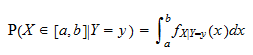for any interval.

In the definition above the quantity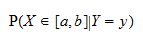is the conditional probability thatwill belong to the interval, given that.

## How to derive it

In order to derive the conditional pdf of a continuous random variable given the realization of another one, we need to know their joint probability density function (see this glossary entry to understand how joint pdfs work).

Suppose that we are told that two continuous random variablesandhave joint probability density function.

Then, we are also told that the realization ofhas been observed and, wheredenotes the observed realization.

How do we compute the conditional probability density function ofso as to take the new information into account?

This is done in two steps:

1. first, we compute the marginal density ofby integrating the joint density: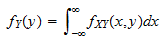2. then, we use the conditional density formula: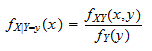## Example

Let's make an example.

Suppose that the joint probability density function ofandis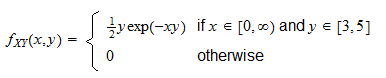The support of(i.e., the set of its possible realizations) isWhen, the marginal pdf ofis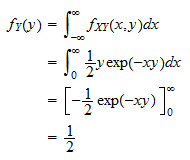When, the marginal pdf ofisbecauseand its integral is zero.

By putting the two pieces together, we obtain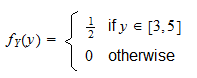Thus, the conditional pdf ofgivenis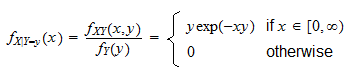Note that we do not need to worry about division by zero (i.e., the case when) because the realization ofalways belongs to the support ofand, as a consequence,.

## How it is used to build joint densities

We have just explained how to derive a conditional pdf from a joint pdf, but things can be done also the other way around: if we are given the marginal pdfand the conditional, then the joint distribution can be derived by performing a simple multiplication: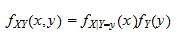## More details

More details about the conditional probability density function can be found in the lecture entitled Conditional probability distributions.

## Keep reading the glossary

Previous entry: Binomial coefficient

Next entry: Conditional probability mass function